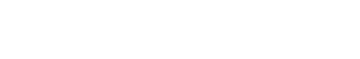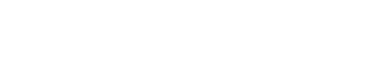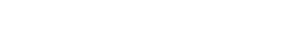# Multi-layer Perceptron Using Python

In this tutorial, we will study multi-layer perceptron using Python. In our previous post, Implementation of Perceptron Algorithm using Python, we learned about single-layer Perceptron, which is the first step towards learning Neural Network.

It is a model inspired by brain, it follows the concept of neurons present in our brain. Several inputs are being sent to a neuron along with some weights, then for a corresponding value neuron fires depending upon the threshold being set in that neuron. For understanding the theory of Perceptron please go through our article on The Perceptron: A theoretical approach. Now, what is the need of Multi-layer Perceptron! To solve more complex problems or to make our machine learn more complex stuff like human faces in an image is a very complex stuff for a machine. Thus we require multiple layers in our Neural Network to solve problem more efficiently. We will use only 2 layers in this algorithm.

### Algorithm of Multi-layer Perceptron

Its algorithm is divided into three phases

• Initialization phase : In this phase, we set the weights to any random values. They can be positive or negative.
• Training phase:
• For T iterations
• For each input vector
• Forward Propagation:
• Compute the activation of each neuron ‘j’ in the hidden layers using:• Compute through layers until we get to output layer, having activation function:
• Backward Propagation:
• Error at the output:• Error in hidden layers:• Update output layer weights:• Update hidden layer weights:• Recall Phase: Use Forward Propagation that we have used above

# Implementation of Multi-layer Perceptron Algorithm

## Layer Data Generator

It generates random weights for both first layer and hidden layer.

In :
```from numpy import exp, array, random, dot, round
from sklearn.utils import shuffle

class Create_Layer_Data():
def __init__(self, neurons, inputs_per_neuron):
self.weights = 2 * random.random((inputs_per_neuron, neurons)) -1
```

## Sigmoid Method

Since we don’t the output of first layer, which will be input for our second layer (hidden layer), we uses Sigmoid function for normalising the values, that is, for any value the result will lie between 0 and 1.

## Deriving Sigmoid Derivative

Since we don’t know in which direction the weight should move Positive (+) or Negative (-), to resolve this problem we use Sigmoid derivative, which tells us whether it is moving away from local minima or towards it, thus by using that we decide what to change in weights.

## Training our Multi-layer Perceptron

For faster and better learning of a Neural Network, especially when we have less data, we shuffle the position of input with respect to outputs for each iteration or epoch.

In :
```class Assign_Layer_Data():
def __init__(self, layer1, layer2):
self.layer1 = layer1  #assigning values genrated from class Create_Layer_Data to the variables of this class
self.layer2 = layer2

# this functoin represents Sigmoid Function
def sigmoid(self, x):
return 1 / (1 + exp(-x))

#Sigmoid Derivative
def sder(self, func_s):
return func_s * (1 - func_s)

# Training our data
def train(self, inputs, outputs, number_of_training_iterations):
for iteration in range(number_of_training_iterations):
# Shuffle positions of inputs and outputs, for fast learning
inputs, outputs = shuffle(inputs, outputs, random_state=0)

# Outputs of layer1 and layer2 are gnerated by predict() method
out_layer1, out_layer2 = self.predict(inputs)

# Calculating the error for layer 2
layer2_error = outputs - out_layer2
layer2_delta = layer2_error * self.sder(out_layer2)

# Calculating the error for layer 1
layer1_error = layer2_delta.dot(self.layer2.weights.T)
layer1_delta = layer1_error * self.sder(out_layer1)

# By what value we will update Weights
layer1_update = inputs.T.dot(layer1_delta)
layer2_update = out_layer1.T.dot(layer2_delta)

# Updating Weights
self.layer1.weights += layer1_update
self.layer2.weights += layer2_update

# Generating output for all layers of neural network and predicting result for our test data
def predict(self, inputs):
output_from_layer1 = self.sigmoid(dot(inputs, self.layer1.weights))
output_from_layer2 = self.sigmoid(dot(output_from_layer1, self.layer2.weights))
return output_from_layer1, output_from_layer2

def print_weights(self):
print ("Layer-1 using 4 Neurons along with 3 Inputs")
print (self.layer1.weights)
print ("Layer-2 using 1 Neuron along with 4 Inputs")
print (self.layer2.weights)
```

## Main() Method

For input data we have use data of A xor B xor C

In :
```if __name__ == "__main__":

#Seeding random number generator
random.seed(1)

# Creating layer-1 consisting 4 Neurons getting 3 inputs each
layer1 = Create_Layer_Data(4, 3)

# Creating layer-2 consisting 1 Neuron getting 4 inputs
layer2 = Create_Layer_Data(1, 4)

# Combining layers to create our Multi-layer Perceptron (Neural Network)
neural_network = Assign_Layer_Data(layer1, layer2)

print ("Initial Data: ")
neural_network.print_weights()

inputs = array([[0, 0, 0], [0, 0, 1], [0, 1, 0], [0, 1, 1], [1, 0, 0], [1, 0, 1], [1, 1, 0]])
outputs = array([[0, 1, 1, 0, 1, 0, 0]]).T

# Train the neural network using the training set.
# Do it 999 times and make small adjustments each time.
epoch = 999
neural_network.train(inputs, outputs, epoch)

print ("Weights after Training")
neural_network.print_weights()

# Test the neural network with a new situation.
print ("Testing our data [1, 1, 0] -> ?: ")
hidden_state, output = neural_network.predict(array([1, 1, 1]))
print (round(output))
```
```Initial Data:
Layer-1 using 4 Neurons along with 3 Inputs
[[-0.16595599  0.44064899 -0.99977125 -0.39533485]
[-0.70648822 -0.81532281 -0.62747958 -0.30887855]
[-0.20646505  0.07763347 -0.16161097  0.370439  ]]
Layer-2 using 1 Neuron along with 4 Inputs
[[-0.5910955 ]
[ 0.75623487]
[-0.94522481]
[ 0.34093502]]
Weights after Training
Layer-1 using 4 Neurons along with 3 Inputs
[[ 0.27224656  3.35304332 -3.32757171 -6.26780686]
[-0.59641049 -6.70331455 -3.41582688  2.83784895]
[-2.30176433  3.77833716  6.58239555  3.20060645]]
Layer-2 using 1 Neuron along with 4 Inputs
[[-7.54614917]
[ 7.3857822 ]
[-9.92062662]
[ 6.02513569]]
Testing our data [1, 1, 0] -> ?:
[1.]
```

Hence we got the right result corresponding to Input vector that we have supplied.

Above post gave us an insight that how we can create multiple layers in a Neural Network and how it does the learning. In our next post we will learn about Multiple other activation functions like tan(h) with their uses in Neural Network.
So stay tuned and keep learning!!!